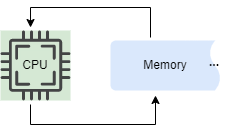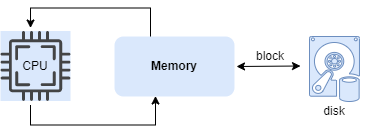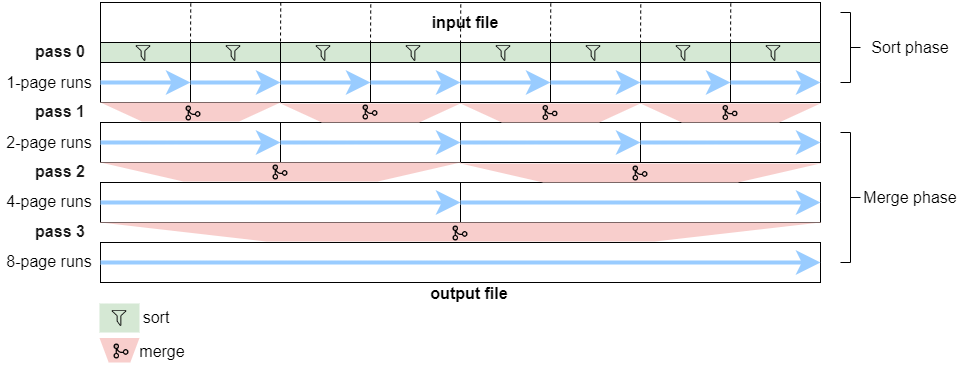# External Mergesort: part one

### Introduction

This article will examine one interesting question which I came across when reading the book Programming Pearls. This question simply goes like this: How to sort a large disk file? The disk file has so much data that it cannot all fit into the main memory. I considered this algorithm question for a while; but noticed that all the classic sorting algorithms, like Quick sort and merge sort, can’t solve it easily. Then I did some research about it, and I will share what I learned in this article. I believe you can solve this problem as well, after reading this article.

### Background of External Algorithm

Traditionally, computer scientists analyze the running time of an algorithm by counting the number of executed instructions, which is usually expressed as a function of the input size n, like the well-known Big O notation. This kind of algorithm complexity analysis is based on the Random access machine(RAM) model, which defines the following assumptions:

• A machine with an unbounded amount of available memory;
• Any desired memory location can be accessed in unit time;
• Every instruction takes the same amount of time.This model works well when the amount of memory that the algorithm needs to use is smaller than the amount of memory in the computer running the code.

But in the real world, some applications need to process data that are too large to fit into a computer’s main memory at once. And the algorithms used to solve such problems are called external memory algorithms or disk-based algorithms; since the input data are stored on an external memory storage device.

Instead of the RAM model, the external memory algorithm is analyzed based on the external memory model. The model consists of a CPU processor with an internal memory of bounded size, connected to an unbounded external memory. One I/O operation consists of moving a block of contiguous elements from external to internal memory(this is called page cache and is managed by the kernel.).Compared with the main memory, the access speed of external memory is slower by several orders of magnitude, even though modern storage techniques such as SSD are already adopted. Thus, for external algorithms, the bottleneck of the performance is disk IO speed instead of the CPU cycles.

In this section, we examined the computational model of the external memory algorithms. Next, let’s review one typical type of external memory algorithm: external sorting.

### External Mergesort

Similar to the traditional internal sorting algorithms, several different solutions are available for external sorting. In this article, I will focus on external mergesort.

External mergesort is a straightforward generalization of internal mergesort. The algorithm starts by repeatedly loading M input items into the memory(since the memory buffer size is limited, can only store M input items at once), sorting them, and writing them back out to disk. This step divides the input file into some(or many, if the input file is very large) sorted runs, each with M items sorted. Then the algorithm repeatedly merges multiple sorted runs, until only a single sorted run containing all the input data remains.

Let’s use the following example model to analyze this algorithm. First of all, assume the memory buffer is limited, and the size is one page. And the input file size is 8 pages. External mergesort can be divided into two phases: sort phase and merge phase.Sort phase:

• Divide the entire input file into 8 groups, each group size is one page(memory buffer capacity).
• Load each page into the memory, sort the items in the memory buffer(with an internal sorting algorithm), and save the sorted items in a temporary sub-file. Each sub-file is called a run.

At the end of the sort phase, 8 temporary sorted 1-page runs will be created. This step can be marked as pass 0.

Merge phase:

The 8 sorted runs in pass 0 will be merged into a single sorted file with 3 more passes.

• pass 1: Perform 4 runs for the merge.
• Run 1: Merge the first two small 1-page runs into a big 2-page run. This merging step goes as follows:
• Read the first two sorted sub-files (one item from each file).
• Find the smaller item, output it in the new sub-file, and the appropriate input sub-file is advanced. Repeat this cycle until the input sub-file is completed. This routine’s logic is the same as the internal mergesort algorithm.
• Run 2: Merge the next two 1-page runs into a 2-page run.
• Run 3 and 4: follow the same process.
• At the end of pass 1, 4 temporary sorted 2-page runs will be created.
• pass 2: Perform 2 runs for the merge.
• At the end of pass 2, 2 temporary sorted 4-page runs will be created.
• pass 3: Perform 1 run for the merge.
• At the end of pass 3, the final 8-page run containing all the sorted items will be created.

Note: the above process may seem complicated at the first sight, but the logic is nearly the same as the internal merge sort. The only difference is internal merging is based on memory buffers, while external merging is based on disk files, and needs reading the item from disk to memory.

Since we keep merging two small sub-files into a big one with doubled size, the above algorithm can be called two-way external merge sorting. We can generalize the idea to multi-way external merge sorting, which merges M runs into one.

Next, let’s analyze its complexity. Suppose the input file has N items and each page consists of B items. And M denotes the number of ways used in the merge phase, thus the number of passes should be: logM(N/B) + 1, where plus one means the first pass in the sort phase. And each pass, each item is read and written once from and to the disk file. So the total number of disk I/O is: 2N*(logM(N/B) + 1)

### Summary

In this article, we examined the abstract computational model of the external memory algorithm and analyzed the details of the external mergesort algorithm. Next article, let’s implement the code and evaluate the performance.